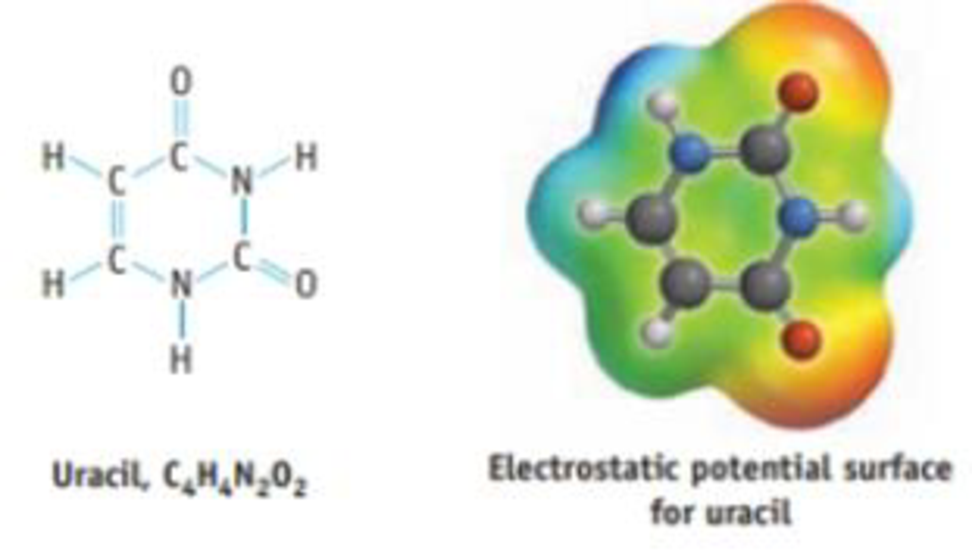Chapter 8, Problem 92IL

Chapter
Section
Textbook Problem

Uracil is one of the bases in RNA, a close relative of DNA.(a) What are the values of the O—C—N and C—N—H angles? (b) There are two carbon-carbon bonds in the molecule. Which is predicted to be shorter? (c) If a proton (H+) attacks the molecule decide on the basis of the electrostatic potential surface to which atom or atoms it could be attached.

(a)

Interpretation Introduction

Interpretation:

The values of the OCN and CNH angles has to be determined.

Concept introduction:

• In VSEPR, the geometry of the molecule is explained based on minimizing electrostatic repulsion between the molecules’ valence electrons around a central atom
• Bond angle is the angle between two bonds of a molecule and it is determined based on the electron-domain geometry.

[Bond angles: tetrahedral = 109.5o, trigonal planar = 120o, T-shape = 90o]

Electron geometry is the shape of a molecule predicted by considering both bond electron pairs and lone pair of electrons.

Molecular geometry is the shape of a molecule predicted by considering only bond pair of electrons.

Explanation

The given compound is Uracil.

Consider the OCN bond in the six-membered ring. The central atom carbon is surrounded by nitrogen, carbon and oxygen. The geometry around the carbon atom will be trigonal planar and hence the bond angle will be approximately 120°

(b)

Interpretation Introduction

Interpretation:

The shorter carbon-carbon bonds in the molecule have to be determined.

Concept introduction:

Bond order: It is the measure of number of electron pairs shared between two atoms.

Bondorder=12(NumberofelectronsinbondoingMOs-NumberofelectronsinantibondingMOs)

Bond length is inversely proportional to the bond order.

(c)

Interpretation Introduction

Interpretation:

The atom on which a proton attacks the molecule has to be determined.

Concept introduction:

Distribution of electrons in a molecule can be shown using electrostatic potential maps three dimensionally. It is also known as electrostatic energy maps. Distributions of charge help to know the interaction of molecule with one another.

Red color in the electrostatic potential map shows that electrons spends more time in that region whereas the blue color shows that electrons spends less time in that region. Green area represents that electrons spend moderate amount of time.

Still sussing out bartleby?

Check out a sample textbook solution.

See a sample solution

The Solution to Your Study Problems

Bartleby provides explanations to thousands of textbook problems written by our experts, many with advanced degrees!

Get Started

The most abundant mineral in the body is iron. T F

Nutrition: Concepts and Controversies - Standalone book (MindTap Course List)

What does density describe?

An Introduction to Physical Science

List the differences between mitosis and meiosis in the following chart:

Human Heredity: Principles and Issues (MindTap Course List)

Do the same types of gene control operate in bacterial cells and eukaryotic cells?

Biology: The Unity and Diversity of Life (MindTap Course List)